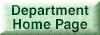### Probability Models and Applications (ERG2040C)

Student/Faculty Expectations on Teaching and Learning Student/Faculty Expectations on Teaching and Learning

Instructor: John C.S. Lui

In this course, we cover fundamental principles of probability theory, and to learn how to use this framework (or methodology) to have logical thinking and carry out analysis to computer science and engineering problems.

Note that this course is "conceptual" and "mathematical" in nature. Students need to spend the time to read the textbook, do the homework, attend the lectures and tutorials so to understand and keep pace with the expectation of this course.

Teaching Assistants (HSH Eng. Bldg, Room 120):

Textbook: A First Course in Probability (8th Edition), by Sheldon Ross

• Homework: 25%;
• Programming or simulation: 25%;
• Examination: 50% (note: you need to get at least 25% in the exam to pass the course)

Policy: Late homework, programming or simulation will NOT be considered.

Course Schedule (2nd term, 2011):

• Lecture:
• Tuesday: 5:30-6:15 PM (LSB LT6);
• Thursday: 12:30-2:15 PM (MMW LT2);
• Tutorial:
• Monday: 12:30-1:15 (LPN LT);
• Monday: 1:30-2:15 PM (LHC G04); TSA;
• Monday: 4:30-5:15 PM (ERB 703); TSA;

Newsgroup:
Please visit the following newsgroup: cuhk.cse.engg2040c, for any announcement. Students can also post comments/questions in the newsgroup.
Students can access the newsgroup via:

• tin (terminal interface),
• thunderbird,
• outlook.

The tentative outline for the course:

• Introduction: why probability theory?
• Combinatorial Analysis
• Axioms of Probability
• Conditional Probability and Independence
• Random Variables: discrete vs. continuous
• Joint Distributed Random Variables
• Expectations and their Properties
• Law of Large Numbers and Limit Theorems
• Markov Processes (*)
• Maximum Likelihood and Hypothesis Testing (*)
• Monte Carlo Simulation

Lecture Notes (note: all lecture notes are password protected)Preliminary Administrative Information and Mutual ExpectationsIntroduction: why study probability?Combinatorial Analysis (Reading Assignment: Chapter 1)Axioms of Probability (part 1) (Reading Assignment: Chapter 2)Axioms of Probability (part 2) (Reading Assignment: Chapter 2)Conditional Probability (Reading Assignment: Chapter 3)Conditional Probability (Part II) (Reading Assignment: Chapter 3)Random Variables (Part I) (Reading Assignment: Chapter 4)Random Variables (Part II) (Reading Assignment: Chapter 4)Continuous Random Variables (Part I) (Reading Assignment: Chapter 5)Continuous Random Variables (Part II) (Reading Assignment: Chapter 5)Jointly Distributed Random Variables (Part I) (Reading Assignment: Chapter 6)Jointly Distributed Random Variables (Part II) (Reading Assignment: Chapter 6)Limit Theorems (Reading Assignment: Chapter 8)

Tutorial NotesTutorial 1Tutorial 2Tutorial 3Tutorial 4: Monte Carlo SimulationTutorial 5: Monte Carlo Simulation 2 ; Java Program on Monte Carlo Simulation ;Tutorial 6Tutorial 7Tutorial 8Tutorial 9Tutorial 10Tutorial 11Tutorial 12Tutorial 13To be added

Homework (students can submit their homework in the drop box, 10/F, HSH Eng. Bldg)Homework 1 ; Solution to Homework 1Homework 2 ; Solution to Homework 2Homework 3 ; Solution to Homework 3Homework 4 ; Solution to Homework 4Homework 5 ; Solution to Homework 5

ProjectMonte Carlo Simulation ; (Programming Assignment is announced !!!) ; Instruction on how to submit the programming assignment ;